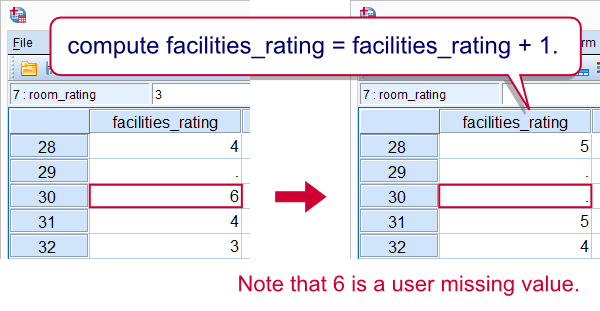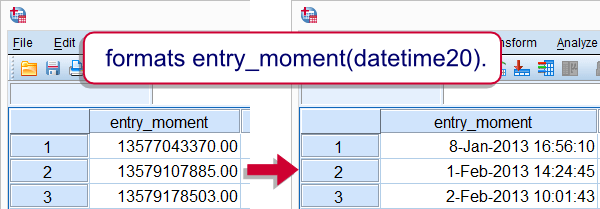SPSS TUTORIALS BASICS ANOVA REGRESSION FACTOR CORRELATION

# SPSS COMPUTE – Simple Tutorial

SPSS COMPUTE command sets the data values for (possibly new) numeric variables and string variables. These values are usually a function (such as MEAN, SUM or something more advanced) of other variables.
This tutorial walks you through doing just that. We'll use hospital.sav,a screenshot of which is shown below.Before proceeding, we'll first set 6 as a user missing value for the last 5 variables. We'll do so by running the syntax below. missing values doctor_rating to facilities_rating (6).

## SPSS COMPUTE Existing Numeric Variable

The simplest COMPUTE example is probably computing an already existing numeric variable. Say we'll add one point to facilities_rating because we feel our respondents were overly negative about it. The syntax below does just that. Note that COMPUTE is a transformation so we also run EXECUTE (“exe.”) in order to see the result.

*Add one point to all values in facilities_rating.

compute facilities_rating = facilities_rating + 1.
exe.Note that 6 does not result in 7. This is because 6 is a user missing value and it's used in a basic numeric function.

## SPSS COMPUTE New Numeric Variable

If the variable that's computed doesn't exit yet, SPSS will create it as a numeric variable having an f format. One of the implications is that we can't directly COMPUTE new string variables but we'll get to that in a minute. We first compute the mean over our 5 ratings but only for cases having at least 3 valid values. Note how this is easily accomplished by using the dot operator.

*Compute mean over 5 rating variables if at least 3 valid values are present.

compute mean_score = mean.3(doctor_rating to facilities_rating).
exe.

## SPSS COMPUTE Existing String Variable

In normal language, COMPUTE usually refers to operations on numbers. In SPSS, however, COMPUTE is used for setting the values of string variables as well. Keep in mind here that you can't use numeric functions on string variables or vice versa. The example below converts surname_prefix to lower case.

*Convert all values in surname_prefix to lower case.

compute surname_prefix = lower(surname_prefix).
exe.

## SPSS COMPUTE New String Variable

SPSS can compute only existing string variables. For new string variables, we must first create new (empty) variables with the STRING command. After doing so, we can set their values with COMPUTE. Like so, the syntax below creates full_name by concatenating the respondents' name components.

*1. Create new (empty) string variable.

string full_name(a25).

*2. COMPUTE full_name with CONCAT and RTRIM.

compute full_name = concat(rtrim(first_name),' ',rtrim(surname_prefix),' ',rtrim(last_name)).
exe.

## SPSS COMPUTE Date, Time and Datetime Variables

COMPUTE can be used for creating new date variables, time variables and datetime variables. This is because these are all numeric variables. However, new numeric variables always have an f format, which is usually not suitable for the aforementioned variables. The way to go here is to first computing the variables by using date functions or basic numeric functions. After doing so, use FORMATS for displaying their values appropriately. The syntax below gives an example.

*1. Compute new datetime variable.

compute entry_moment = entry_date + entry_time.
exe.

*2. Show datetime values (in seconds) as normal dates with times.

formats entry_moment(datetime20).# Tell us what you think!

*Required field. Your comment will show up after approval from a moderator.

# THIS TUTORIAL HAS 9 COMMENTS:

•### By Ruben Geert van den Berg on January 13th, 2019

Hi Erik!

First off, I'll assume that missing values count as 0, ok?

Now the easiest way is to simply create a variable holding the number of 1's over all medicines. Then change 1, 2, 3 and 4 to 1 and leave 0 as 0 with RECODE as in

RECODE myvariable (1 thru hi = 1).

Solutions 1 and 2 below use this.

Solution 3 is rather puzzling but explained in Compute A = B = C.

Solution 4 uses IF which is a very simple but must-know command for questions like these.

Hope that helps!

SPSS tutorials

*Create test data.
data list free/id.
begin data
0 0 0 0 0 0 0 0 0 0 0 0 0 0 0 0 0 0 0 0 0 0 0 0 0 0 0 0 0 0 0 0 0 0 0 0 0 0 0 0 0 0 0 0 0 0 0 0 0 0
0 0 0 0 0 0 0 0 0 0 0 0 0 0 0 0 0 0 0 0 0 0 0 0 0 0 0 0 0 0 0 0 0 0 0 0 0 0 0 0 0 0 0 0 0 0 0 0 0 0
0 0 0 0 0 0 0 0 0 0 0 0 0 0 0 0 0 0 0 0 0 0 0 0 0 0 0 0 0 0 0 0 0 0 0 0 0 0 0 0 0 0 0 0 0 0 0 0 0 0
0 0 0 0 0 0 0 0 0 0 0 0 0 0 0 0 0 0 0 0 0 0 0 0 0 0 0 0 0 0 0 0 0 0 0 0 0 0 0 0 0 0 0 0 0 0 0 0 0 0
end data.

compute id = \$casenum.

set seed 1.
do repeat #vars = chol_1 to chol_4.
compute #vars = rv.bernoulli(0.2).
if(rv.bernoulli(0.05)) #vars = \$sysmis.
if(rv.bernoulli(0.05)) #vars = 2.
end repeat.
execute.

value labels chol_1 to chol_4 0 "Don't use" 1 "Use" 2 "Don't know".

missing values chol_1 to chol_4 (2).

formats all(f3).

*Solution 1.
compute chol_med_1 = sum(chol_1 to chol_4).
recode chol_med_1 (1 thru hi = 1).
execute.

*Solution 2.
count chol_med_2 = chol_1 to chol_4 (1).
recode chol_med_2 (1 thru hi = 1).
execute.

*Solution 3.
compute chol_med_3 = (sum(chol_1 to chol_4) > 0).
execute.

*Solution 4.
compute chol_med_4 = 0.
if(sum(chol_1 to chol_4) > 0) chol_med_4 = 1.
execute.

•### By Sam Adams on April 13th, 2021

I've seen examples of "COMPUTE" that have two equal's signs. I'm thinking they do binary assignments (?) Can't find the examples again and SPSS documentation doesn't have anything. Can you solve the puzzle of what this use is for?

•### By Ruben Geert van den Berg on April 14th, 2021

Hi Sam!

Do you mean something like

COMPUTE V1 = (V2 = V3)?

I covered that in Compute A = B = C.

Hope that helps!

•### By Sisay gemede on August 24th, 2021

Basics of computing variable